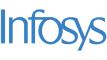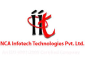New update is available. Click here to update.
Topics

# Prime Time Again

Moderate0/80
Average time to solve is 25m+3 more companies

## Problem statement

You have been given two integers ‘DAY_HOURS’ and ‘PARTS’. Where ‘DAY_HOURS’ is the number of hours in a day and a day can be divided into ‘PARTS’ equal parts. Your task is to find total instances of equivalent prime groups. Prime group refers to the group of elements (hours) which are prime and measure the same equivalent time since the start of the day.

For example, if we consider ‘DAY_HOURS’ to be 24 and ‘PARTS’ to be 2, then the day of total 24 hours is divided into 2 parts ( 1 - 12 ) and ( 13 - 24 ). 5 hours in the first part of the day is equivalent to 17, which is 5 hours into the second part of the day. And since 5 and 17 both are prime, they can be considered as a prime group.

Note:
``````1. Day starts with hour 1 and ends with hour  ‘DAY_HOURS’.

2. Each hour of the prime group should be in a different part of the day.

3. If there is no prime group then return zero.

4. ‘DAY_HOURS’ should be divisible by ‘PARTS’, meaning that the number of hours per part (DAY_HOURS/PARTS)  should be a natural number.
``````

Example:

``````Let ‘DAY_HOURS’ = 20  and ‘PARTS’ = 2

Hence the view of our day would be in the following format:

1  2  3  4  5  6  7  8  9 10      -  Part 1
11 12 13 14 15 16 17 18 19 20     -  Part 2

1-11  Not a prime group because 1 is not prime.
2-12  Not a prime group because 12 is not prime.
3-13  Because both 3 and 13 are prime, it is an equivalent prime group.
4-14  Not a prime group because 4 and 14 are not prime.
5-15  Not a prime group because 15 is not prime.
6-16  Not a prime group, because 6 and 16 are not prime.
7-17  Because both 7 and 17 are prime, it is an equivalent prime group.
8-18  Not a prime group, because 8 and 18 are, is not prime.
9-19  Not a prime group because 9 is not prime.
10-20 Not a prime group because both 10 and 20 are not prime.

Hence there are 2 equivalent prime groups in the above format which are 3-13 and 7-17.
``````
Detailed explanation ( Input/output format, Notes, Images )
Constraints:
``````1 <= T <= 100
10 <= DAY_HOURS <= 5 * 10^3
2 <= PARTS <= 10^3

Time Limit: 1 second
``````
Sample Input 1:
``````2
36 3
8 2
``````
Sample Output 1:
``````2
1
``````
Explanation of sample input 1:
``````Test Case 1:

36 hour day can divide in such three parts:

1  2  3  4  5  6  7  8  9 10 11 12     -Part 1
13 14 15 16 17 18 19 20 21 22 23 24     -Part 2
25 26 27 28 29 30 31 32 33 34 35 36     -Part 3

1-13-25  Not a prime group because 1, 25 are not prime.
2-14-26  Not a prime group because 14, 25  are not prime.
3-15-27  Not a prime group because 15 is not prime.
4-16-28  Not a prime group because 4, 16, 28 are not prime.
5-17-29  Because 3, 17, 29 all are prime, it is an equivalent prime group.
6-18-30  Not a prime group because 6,18,30 are not prime.
7-19-31  Because 7, 19, 31 all are prime, it is an equivalent prime group.
8-20-32  Not a prime group, because 8, 20, 32 are not prime.
9-21-33  Not a prime group because 9, 21, 33 are not prime.
10-22-34 Not a prime group because 10, 22, 34 are not prime.
11-23-35  Not a prime group because 35 is not prime.
12-24-36 Not a prime group because 12, 24, 26 are not prime.

Hence there are 2 equivalent prime groups in the above format  which is 5-17-29 and 7-19-31

Test case 2:

8 hours a day can divide into 2 such parts (1-4) and (5-8)
Hence only one combination of 3-7 is a prime group because 3 and 7 both are prime and are equivalent hours.
``````
Sample Input 2:
``````2
24 2
49 7
``````
Sample Output 2:
``````3
0
``````Console